# A Reactive Hydromagnetic Internal Heat Generating Fluid Flow Through A Channel

A Reactive Hydromagnetic Internal Heat Generating Fluid Flow Through A Channel

Dept. of Mathematics, Tai Solarin University of Education, Ijagun, Nigeria

Dept. of Mathematics, University of Ilorin, Ilorin, Nigeria

Corresponding Author Email:
anthonyhassan72@yahoo.co.uk
Page:
43-50
|
DOI:
http://doi.org/10.18280/ijht.330306
N/A
|
Accepted:
N/A
|
Published:
30 September 2015
| Citation

OPEN ACCESS

Abstract:

This paper investigates the analytical study of the temperature fluid distribution in a one -dimensional fluid flow under a magnetic field. It studies the effect of internal heat generation on the entropy generation in an exothermic reactive hydromagnetic fluid flow under Arrhenius kinetics. The fluid is assumed to be incompressible and electrically conducting flowing steadily through a channel with isothermal wall temperature. The solution is obtained taking into account a supplementary term in energy equation due to internal heat generation using the traditional perturbation method. Thermo- physical aspects of the flow are presented and discussed.

Keywords:

Internal heat generation, Arrhenius kinetics, Entropy generation, Hydromagnetic fluid.

1. Introduction

Over the past few decades, studies relating to flow of combustible fluids are on the increase due to its numerous applications in many industrial and engineering processes like; nuclear reactor, bomb detonation and petro-chemical fluid flows in refineries to mention just a few. In fact, the literature is very rich on studies relating to combustible fluid flows (see ref. [1-5]). In the present investigation, our attention is focused on the effect of internal heat generation on the fluid flow where in the papers [1-5], analysis was done in the absence of internal heat generation.

After an exhaustive survey of literature, it is observed that studies relating to the second law analysis of reactive internal heat generating hydromagnetic fluid flow through a channel with isothermal wall temperature have not been studied. However, from application point of view, recent studies have shown that for fluid flows involving exothermic/ endothermic reactions, fire and combustion; the effect of internal heat generation cannot be neglected [6-18].

Therefore, the purpose of this paper is to investigate the effect of this all-important flow property on the fluid flow, the present study is relevant in several applications in the metallurgical and petro-chemical engineering.  The problem is strongly nonlinear involving exponential nonlinearity. Hence, analytical solution will be obtained using the traditional perturbation technique. The rest of the paper is organised as follows; in section 2 the problem is formulated. In section 3, the problem is solved together with entropy analysis. Results are presented and discussed in section 4 while section 5 gives some concluding remarks.

2. Model Formulation

Consider the steady flow of a reactive, incompressible and electrically conducting fluid flowing through a channel between two parallel plates with isothermal wall temperature under the influence of a transverse magnetic field strength B0 as shown in Figure 1. The internal heat generation term Q in this problem is assumed to be a linear function of temperature [19-20].

## 0.png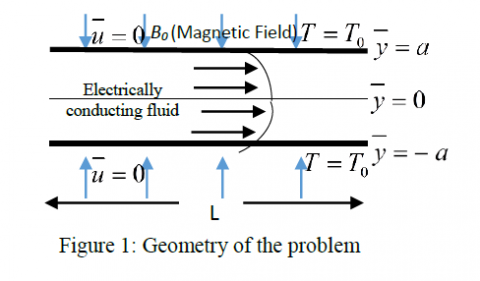$Q=Q_{0}\left(\bar{T}-T_{0}\right)$    (1)

Where Q0 is dimensional heat generation coefficient, T is the fluid temperature and To represent wall temperature.

Neglecting the consumption of the reactant, the continuity, momentum and energy equations governing the flow in dimensionless form may be written as:

$\frac{\partial u}{\partial x}+\frac{\partial v}{\partial y}=0$    (2)

$\varepsilon^{2} \operatorname{Re}\left[u \frac{\partial u}{\partial x}+v \frac{\partial u}{\partial y}\right]=-\frac{\partial p}{\partial x}+\mu\left[\left(\varepsilon^{2} \frac{\partial^{2} u}{\partial x^{2}}+\frac{\partial^{2} u}{\partial y^{2}}\right)\right]-H a^{2} u$      (3)

$\varepsilon^{4} \operatorname{Re}\left[u \frac{\partial v}{\partial x}+v \frac{\partial v}{\partial y}\right]=-\frac{\partial p}{\partial y}+\mu\left(\varepsilon^{2} \frac{\partial^{2} v}{\partial y^{2}}+\varepsilon^{4} \frac{\partial^{2} v}{\partial x^{2}}\right)$     (4)

$\varepsilon^{2} P e\left[u \frac{\partial T}{\partial x}+v \frac{\partial T}{\partial y}\right]=\varepsilon^{2} \frac{\partial^{2} T}{\partial x^{2}}+\frac{\partial^{2} T}{\partial y^{2}}+\lambda e^{\frac{T}{l+\delta T}}+\varphi+B r H a^{2} u^{2}+\beta_{0} T$      (5)

Where $\varphi=B r\left[2 \varepsilon^{2}\left(\frac{\partial u}{\partial x}\right)^{2}+2 \varepsilon^{2}\left(\frac{\partial v}{\partial y}\right)^{2}+\left(\frac{\partial u}{\partial y}+\varepsilon^{2} \frac{\partial v}{\partial x}\right)^{2}\right]$

Additional term in the energy equation is due to internal heat generation. Here, the following non-dimensional variables and parameters have been used

$y=\frac{\bar{y}}{\varepsilon L}, x=\frac{\bar{x}}{L}, u=\frac{\bar{u}}{U}, v=\frac{\bar{v}}{\varepsilon U}, \varepsilon=\frac{a}{L}$

$T=\frac{E\left(\bar{T}-T_{0}\right)}{R T_{0}^{2}}, P=\frac{\varepsilon^{2} L \bar{P}}{\mu U}, B r=\frac{E \mu U^{2}}{k R T_{0}^{2}}$

$\lambda=\frac{Q E A a^{2} C_{0}}{k R T_{0}^{2}} e^{-\frac{E}{R T_{0}}}, \operatorname{Re}=\frac{\rho U L}{\mu}, \delta=\frac{R T_{0}}{E}$

$G=-\frac{d P}{d x}, \gamma=\frac{\mu U^{2} e^{\frac{E}{R T_{0}}}}{Q A a^{2} C_{0}}, H a^{2}=\frac{\sigma B_{0}^{2} a^{2}}{\mu}$

$P e=\frac{\rho C_{p} U L}{k}, \beta_{0}=\frac{Q_{0} a^{2}}{k}, \beta=\frac{Q_{0} R T_{0}^{2} e^{\frac{E}{R T_{0}}}}{Q E C_{0} A}$   (6)

Where L is the channel characteristic length, a is channel half width, E is activation energy, G is constant axial pressure gradient and R is the universal gas constant. Ha represents Hartmann number, Be is Bejan number, Br is Brinkman number, Pe is Peclet Number and Re is Reynolds number. Co is reactant species initial concentration, A is reaction rate constant, ρ is fluid density, ε is channel aspect ratio, μ is fluid viscosity, v is normal velocity, U is mean velocity, u is axial velocity, Cp is specific heat at constant pressure and k thermal conductivity coefficient. Also, σ represents electrical conductivity; λ is Frank-Kamenettski parameter, γ is viscous heating parameter, β and β0 are heat generation parameters and δ is the activation energy parameter.

Since the channel aspect ratio is small (0 < ε << 1), the lubrication approximation based on an asymptotic simplification of the governing equations (1)-(5) is invoked and we obtained

$\frac{d^{2} u}{d y^{2}}-H a^{2} u=-G$    (7)

$\frac{d^{2} T}{d y^{2}}+\lambda\left[e^{\frac{T}{1+\delta T}}+\gamma\left(\left(\frac{d u}{d y}\right)^{2}+H a^{2} u^{2}\right)+\beta T\right]=0$     (8)

Together with the boundary conditions

$\frac{d T}{d y}(0)=0=\frac{d u}{d y}(0)$

$u(1)=T(1)=0$      (9)

3.1 Method of solution

3.1 Method of solution

Solving (7) with the appropriate boundary conditions, one obtain

$u(y)=\frac{G}{H a^{2}}\left(1-\frac{\cosh (H a y)}{\cosh (H a)}\right)$    (10)

With (10) in (8), it will be convenient to assume a series solution in the form

$T(y)=\sum_{n=0}^{\infty} \lambda^{n} T_{n}(y)$     (11)

Where 0 < λ << 1

Clearly, $e^{\frac{T}{1+\delta T}}$ can be Taylor’s series expanded, using (11) in (8) and equating the orders of λ, we get the following:

$\mathrm{O}\left(\lambda^{0}\right): \frac{d^{2} T_{0}}{d y^{2}}=0$,

Such that,$T_{0}^{\prime}(0)=0, T_{0}(1)=0$     (12)

$\mathrm{O}\left(\lambda^{1}\right): \frac{d^{2} T_{1}}{d y^{2}}+e^{\frac{T_{0}}{1+\delta T_{0}}}+\gamma\left[\left(\frac{d u}{d y}\right)^{2}+H a^{2} u^{2}\right]+\beta T_{0}=0$      (13)

Such that

$\mathrm{O}\left(\lambda^{2}\right): \frac{d^{2} T_{2}}{d y^{2}}+\frac{e^{\frac{T_{0}}{1+\delta T_{0}}} T_{1}}{\left(1+\delta T_{0}\right)^{2}}+\beta T_{1}=0$     (14)

Such that $T_{2}^{\prime}(0)=0, T_{2}(1)=0$

$\mathrm{O}\left(\lambda^{3}\right): \frac{d^{2} T_{3}}{d y^{2}}+\frac{e^{\frac{T_{0}}{1+\delta T_{0}}}}{2\left(1+\delta T_{0}\right)^{4}}\left[\begin{array}{l}T_{1}^{2}-2 \delta T_{1}^{2} \\ -2 \delta^{2} T_{0} T_{1}^{2}+2 T_{2} \\ +4 \delta T_{0} T_{2}+2 \delta^{2} T_{0}^{2} T_{2}\end{array}\right]+\beta T_{2}=0$    (15)

Such that $T_{3}^{\prime}(0)=0, T_{3}(1)=0$.

Solving (12) to (15), then (11) can be written as

## 1.png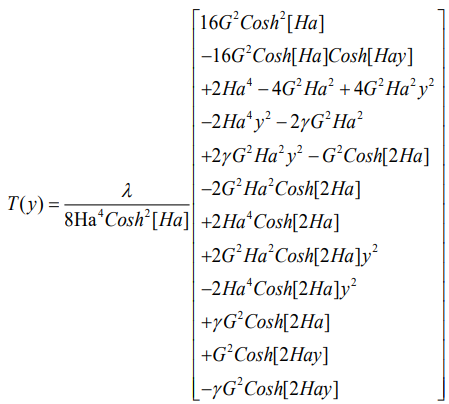## 2.png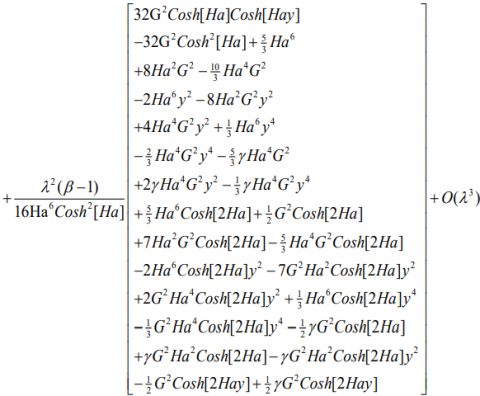(16)

Other physical interest includes the rate of heat transfer across the channel which is given by

$N u=-\left.\frac{d T}{d y}\right|_{y=1}$  (17)

Where Nu is the Nusselt number.

And the Skin friction (Cf ) at the upper wall of the channel is given by

$C_{f}=\left.\frac{d u}{d y}\right|_{y=1}$     (18)

3.2 Entropy generation analysis

Inherent irreversibility in a channel flow arises due to exchange of energy and momentum within the fluid and the solid boundaries. The entropy production is due to heat transfer and the combined effects of fluid friction and Joules dissipation. Following , the general equation for the entropy generation per unit volume in the presence of a magnetic field is given by:

$S^{m}=\frac{k}{T_{0}^{2}}\left(\frac{d \bar{T}}{d \bar{y}}\right)^{2}+\frac{\mu}{T_{0}}\left(\frac{d \bar{u}}{d \bar{y}}\right)^{2}+\frac{\sigma B_{0}^{2} \bar{u}^{-2}}{T_{0}}$     (19)

The first term in (19) is the irreversibility due to heat transfer, the second term is the entropy generation due to viscous dissipation and the third term is the local entropy generation due to the effect of the magnetic field.

We express the entropy generation number in dimensionless form using the existing non-dimensional variables and parameter as:

$N_{s}=\frac{S^{m} a^{2} E^{2}}{k R^{2} T_{0}^{2}}=\left(\frac{d T}{d y}\right)^{2}+\frac{B r}{\Omega}\left[\left(\frac{d u}{d y}\right)^{2}+H a^{2} u^{2}\right]$    (20)

The first term, $\left(\frac{d T}{d y}\right)^{2}$ is assigned N1 which is the irreversibility due to heat transfer and the second term, $\frac{B r}{\Omega}\left[\left(\frac{d u}{d y}\right)^{2}+H a^{2} u^{2}\right]$ referred to as N2 is the entropy generation due to the combined effects of viscous dissipation and magnetic field where $\Omega=\frac{R T_{0}}{E}$ is  the wall temperature parameter.

We defined

$\varphi=\frac{N_{2}}{N_{1}}$    (21)

as the irreversibility distribution ratio.  Relation (21) shows that heat transfer dominates when $\phi>1$ and fluid friction dominates when ϕ > 1. This is used to determine the contribution of heat transfer in many engineering designs.

As an alternative to irreversibility parameter, the Bejan number (Be) is defined as

$B e=\frac{N_{1}}{N_{s}}=\frac{1}{1+\varphi}$

Where $0 \leq B e \leq 1$    (22)

4. Discussion of Results

In this section, the effect of internal heat generation together with other important flow parameter on the reactive hydro magnetic fluid flow through a channel with isothermal wall temperature are presented and discussed.

Table 1. Computation of the entropy generation analysis for various values of internal heat generation parameter

 y $N_{1}$ $N_{2}$ $B e$ β = 0 β =0.5 β = 1 β=0 β=0.5 β = 1 -1 0.0068 0.0070 0.0072 0.2320 0.0283 0.0292 0.0301 -0.75 0.0029 0.0030 0.0032 0.1240 0.0228 0.0237 0.0248 -0.5 0.0010 0.0010 0.0011 0.0746 0.0129 0.0136 0.0143 -0.25 0.0002 0.0002 0.0002 0.0547 0.0036 0.0038 0.0041 0 0 0 0 0.0496 0 0 0 0.25 0.0002 0.0002 0.0002 0.0547 0.0036 0.0038 0.0041 0.5 0.0010 0.0010 0.0011 0.0746 0.0129 0.0136 0.0143 0.75 0.0029 0.0030 0.0032 0.1240 0.0228 0.0237 0.0248 1 0.0068 0.0070 0.0072 0.2320 0.0283 0.0292 0.0301

The table 1 shows the effect of internal heat generation in the fluid flow, as the internal heat generation parameter (β) increases, it is also observed that the fluid friction dominates as β lies between 0 and 1.

## 2.png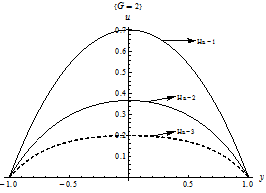Figure 2. Velocity Profile for several values of Ha

Figure 2 shows the plot of velocity profile for variation in Hartmann number (Ha). As observed, maximum velocity occurs at the minimum value of the parameter. Further increase in Ha decreases the flow velocity maximum; this is due to the presence of Lorentz forces which has retarding effects on fluid flow when placed across the flow channel.

## 3.png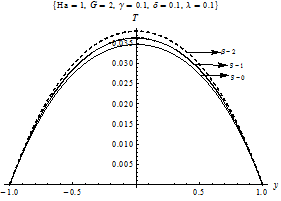Figure 3. Temperature Profile for several values of β

## 4.pngFigure 4. Temperature Profile for several values of δ

Figure 3 depicts the temperature profile for variations in internal heat generation parameter (β). From the plot, it is observed that an increase in the internal heat absorption increases the fluid temperature within the channel. It is important to note that internal heat generation which shows an increase in fluid temperature is due to reduction in the thermal conductivity of the fluid.

Figure 4 shows the effect of activation energy (δ) on the temperature distribution within the channel. The result shows that an increase in δ decreases the fluid temperature due to rise in fluid viscosity. This is physically true due to the fact that higher activation energy means slower chemical reaction due to the energy difference between the reactants and the energy needed for the reaction to occur.

## 5.pngFigure 5. Temperature Profile for various values of γ

Figure 5 presents the temperature profile for various values of the viscous heating parameter (γ). From the result, an increase in viscous heating increases the temperature distribution within the channel. This is due to rise in kinetic energy of the fluid.

## 6.png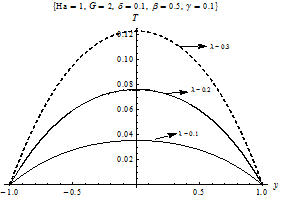Figure 6. Temperature Profile for various values of λ

Figure 6 shows the plot of temperature profile for various values of Frank-Kamenettski parameter (λ). Increasing values of the Frank-Kamenettski parameter (λ) leads to an increase in the temperature of the fluid due to the increase in the initial concentration of the reactant.

Figures 7-10 show the entropy generation rate for various parametric values. On a general note, the entropy generation rate is maximum at the plate surfaces and minimum around the core region of the channel. The entropy generation rate increases with increasing values of internal heat generation parameter (β), Frank-Kamenettski parameter (λ) and the group parameter (Br Ω–1) in the thermodynamic performance of the flow system. While in figure 8, the entropy generation rate decreases with the increasing value of the Hartmann number (Ha). This is true because the rate of disorder is reduced with increase in magnetic field intensity.

## 7.png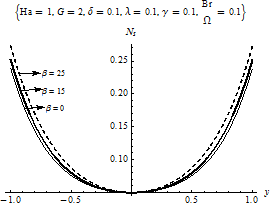Figure 7. Entropy generation rate for various values of β

## 8.png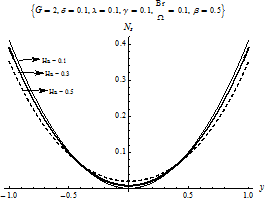Figure 8. Entropy generation rate for various values of Ha

## 9.png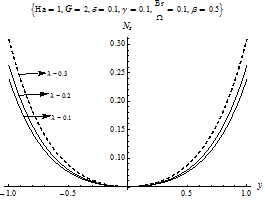Figure 9. Entropy generation rate for various values of λ

## 10.png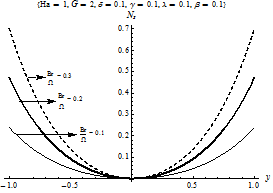Figure 10. Entropy generation rate for various values of Br/Ω

## 11.png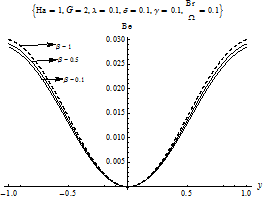Figure 11. Bejan number for various values of β

## 12.png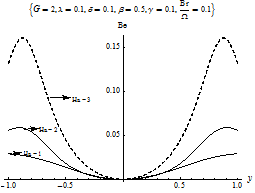Figure 12. Bejan number for various values of Ha

## 13.pngFigure 13. Bejan number for various values of λ

Figures 11–13 display the Bejan (Be) number for various parametric values in the channel width. The general observation is that the fluid friction irreversibility dominates at the channel core region while the heat transfer irreversibility dominates at both lower and upper wall surfaces. The dominant effect of heat transfer irreversibility of the plate increases with increasing values of internal heat generation parameter (β), Frank-Kamenettski parameter (λ) and the magnetic Hartmann number (Ha).

## 14.png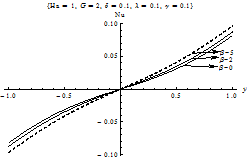Figure 14. Rate of heat transfer

## 15.png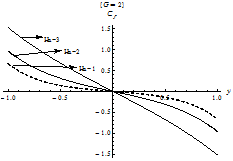Figure 15. Skin Friction of the channel

Figure 14 shows the rate of heat transfer for various values of internal heat generation parameter (β). It is observed that the rate of heat transfer increases as the internal heat generation parameter (β) increases in the fluid flow while figure 15 shows the skin friction of the fluid flow. The fluid skin friction increases as the Hartmann number (Ha) increases.

5. Conclusion

In this present work, we have investigated the effects of internal heat generation on the reactive flow of an electrically conducting fluid through a channel with isothermal wall temperature. The analytical solutions of the nonlinear dimensionless equations governing the fluid flow are obtained using traditional perturbation technique.  Results of the computation shows the effect of internal heat generation on the thermal conductivity of the fluid.

Nomenclature
 L Channel characteristic length E Activation energy G Constant axial pressure gradient R Universal gas constant Ha Hartmann number Bo Magnetic field strength Be Bejan number A Reaction rate constant Br Brinkman number Nu Wall heat flux (Nusselt number) ρ Fluid density σ Electrically conductivity ε Channel aspect ratio μ Viscosity λ Frank-Kamenettski parameter α critical exponent δ Activation energy parameter U Mean velocity (ms-1) γ Viscous heating parameter Pe Peclet Number ϕ Irreversibility distribution ratio Re Reynolds number β Heat generation parameter Ω Wall temperature parameter T Temperature (k) To Wall temperature (k) Co Reactant species initial concentration v Normal velocity (ms-1) Qo Dimensional heat generation coefficient Q Heat generation term (W) Cp Specific heat at constant pressure a Channel half width (m) k Thermal conductivity coefficient (Wm-1k-1) u Axial velocity (ms-1) Cf Skin friction x Horizontal coordinates system (m) y Vertical coordinate system (m)
References

1. S. O. Adesanya and R. O. Ayeni, Heat Transfer to Poiseuille Flow of a Reacting Pressure and Temperature Dependent Viscosity in a Channel, Journal of Modern Mathematics and Statistics, vol. 2, no. 2, pp.  40-44, 2008.

2. S. S. Okoya, Thermal Stability for a Reactive Viscous Flow in a Slab, Mechanics Research Communication, vol.33, pp. 728-733, 2006. DOI: 10.1016/j.mechrescom.2005.11.004.

3. S. S. Okoya, Criticality and Transition for a Steady Reactive Plane Couette Flow of a Viscous Fluid, Mechanics research Communication, vol. 34, pp. 130- 135, 2007. DOI: 10.1016/j. mechrescom.2006.09.006.

4. S. S. Okoya, Disappearance of Criticality for a Reactive Third-grade Fluid with Reynolds’s Model Viscosity in a Flat Channel, International Journal of Non–Linear Mechanics, vol. 46, pp. 1110-1115, 2011. DOI: 10.1016/j.ijnonlinmec.2011.04.008.

5. S.O. Ajadi, A Note on the Thermal Stability of a  Reactive Non–Newtonian Flow in a Cylindrical Pipe, International Communications in Heat and Mass Transfer, vol. 36, pp. 63-68, 2009. DOI: 10.1016/j.icheatmasstransfer.2008.09.005.

6. S. O. Adesanya, Steady Magnetohydrodynamic Visco- Elastic Heat Generating /Absorbing Slip Flow through a Porous Medium with Radiation Effect, International Journal of Heat and Technology, vol. 30, no. 1, pp. 69- 74, 2012.

7. P. M. Patil, P. S. Kulkarni, Effects of Chemical Reaction on Free Convective Flow of a Polar Fluid through  Porous Medium in the Presence of Internal Heat Generation, International Journal of Thermal Sciences, vol. 47, pp. 1043–1054, 2008. DOI: 10.1016/j.ijthermalsci.2007.07.013.

8. C. Chen, On Analytic Solution of MHD Flow and Heat Transfer for Two Types of Viscoelastic Fluid over a Stretching Sheet with Energy Dissipation, Internal Heat Source and Thermal Radiation, International Journal of Heat and Mass Transfer, vol. 53, pp. 4264-4273, 2010. DOI: 10.1016/j.ijheatmasstransfer.2010.05.053.

9. J. M. Jawdat and I. Hashim, Low Prandtl Number  Chaotic Convection in Porous Media with Uniform Internal Heat Generation, International Communications in Heat and Mass Transfer, vol. 37, pp. 629-636,   2010.  DOI: 10.1016/j.icheatmasstransfer.2010.03.011.

10. S. Bagai and C. Nishad, Free Convection in a Non- Newtonian Fluid along a Horizontal Plate Embedded  in a Porous Media with Internal Heat Generation, International Communications in Heat and Mass Transfer, vol. 39, pp. 537-540, 2012. DOI: 10.1016/j.icheatmasstransfer.2012.02.003.

11. A. Bartella and D. A. Nield, On the Rayleigh–Bernard Poiseulle Problem with Internal Heat Generation, International Journal of Thermal Sciences, vol. 57,  1– 16, 2012. DOI: 10.1016/J.IJTHERMALSCI.2012.02.014.

12. V. Marcello, A. Cammi and L. Luzzi, A Generalized Approach to Heat Transfer in Pipe Flow with Internal Heat Generation, Chemical Engineering Science, vol. 65, pp. 1301–1310, 2010. DOI: 10.1016/j.ces.2009.10.004.

13. S. Siddiqa, S. Asghar and M. A. Hossain, Natural Convection Flow over an Inclined Flat Plate with  Internal Heat Generation and Variable Viscosity, Mathematical and Computer Modelling, vol. 52, pp. 1739-1751, 2010. DOI: 10.1016/j.mcm.2010.07.001.

14. C. Chen, Magneto-hydrodynamic Mixed Convection of  a Power–law Fluid Past a Stretching Surface in the Presence of Thermal Radiation and Internal Heat Generation/Absorption, International Journal of Non– Linear Mechanics, vol. 44, pp. 596-603, 2009. DOI: 10.1016/j.ijnonlinmec.2009.02.004.

15. G. K. Perekattu and C. Balaji, On the Onset of Natural Convection in Differentially Heated Shallow Fluid Layers with Internal Heat Generation, International Journal of Heat and Mass Transfer, vol. 52, pp. 4254– 4263, 2009. DOI: 10.1016/j.ijheatmasstransfer.2009.04.006.

16. A. Ben–Nakhi, A. J. Chamkha, Conjugate Natural Convection Around a Finned Pipe in a Square Enclose with Internal Heat Generation, International Journal of Heat and Mass Transfer, vol. 50, pp. 2260-2271, 2007. DOI: 10.1016/j.ijheatmasstransfer.2006.10.036.

17. H. Oztop and E. Bilgen, Natural Convection in Differentially Heated and Partially Divided Square Cavities with Internal Heat Generation, International Journal of Heat and Fluid Flow, vol. 27, pp. 466-475, 2006. DOI: 10.1016/j.ijheatfluidflow.2005.11.003.

18. R. Cortell, Flow and Heat Transfer of a Fluid through a Porous Medium over a Stretching Surface with Internal Heat Generation/Absorption and Suction/Blowing, Fluid Dynamics Research, vol. 37, pp. 231-245, 2005. DOI: 10.1016/j.fluiddyn.2005.05.001.

19. M. F. El-Amin, Combined Effect of Internal Heat Generation and Magnetic Field on Free Convection and Mass Transfer Flow in a Micro Polar Fluid with  Constant Suction, Journal of Magnetism and Magnetic Materials, vol. 270, pp.130–135, 2004. DOI: 10.1016/j.jmmm.2003.08.011.

20. B. K. Jha and A. O. Ajibade, Free Convective Flow of Heat Generating/Absorbing Fluid between Vertical Porous Plates with Periodic Heat Input, International Communications in Heat and Mass Transfer, vol. 36, pp.624–631, 2009. DOI: 10.1016/j.icheatmasstransfer.2009.03.003.

21. L. C. Wood, The Thermodynamics of Fluid System, Oxford University Press, Oxford, 1975.